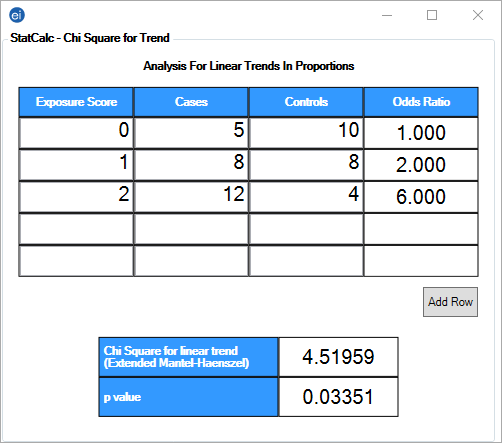# StatCalc: Statistical Calculators

StatCalc

### Chi Square for Trend

The Chi Square for Trend function calculates the odds ratio, chi square for linear trend, and p-value statistics based on the response to an exposure score and whether the patient has become ill. The exposure score is a measured outcome from a study that states the level of exposure the patient received. As an example, 0 = no exposure, 1 = moderate exposure, 2 = significant exposure. Values entered into the Exposure Score column must be numeric. Enter the number of patients who are ill for each exposure score in the Cases column. Enter the number of patients who are not ill for each exposure score in the Controls column. The odds ratio, chi square for linear trend statistic, and p-value for the chi square automatically calculate.Figure 10.13: Chi Square for Trend

In this example, the odds ratio states how likely a patient is to become ill based on the level of exposure. A patient is twice as likely to become ill with moderate levels of exposure (exposure score = 1) and six times as likely to become ill with significant levels of exposure (exposure score = 2) as a patient that has not been exposed (exposure score = 0). The chi square for linear trend and p-value statistics provide a level of significance for the study.

Note: A p-value < .05 is generally considered statistically signficant

Page last reviewed: September 16, 2022, 12:00 pm